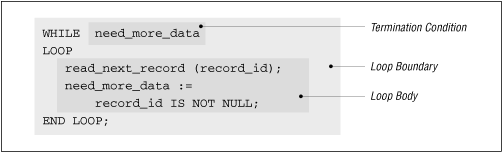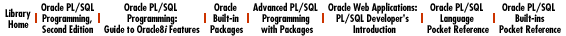home | O'Reilly's CD bookshelfs | FreeBSD | Linux | Cisco | Cisco Exam

# 7. Loops

This chapter explores the iterative control structures of PL/SQL, otherwise known as loops, which let you execute the same code repeatedly. PL/SQL provides three different kinds of loop constructs:

• The simple or infinite loop

• The FOR loop (numeric and cursor)

• The WHILE loop

Each type of loop is designed for a specific purpose with its own nuances, rules for use, and guidelines for high-quality construction. As I explain each of the loops, I will include a table (based on the following one) describing the following properties of the loop:

Property

Description

How the loop is terminated A loop executes code repetitively. How do you make the loop stop executing its body?
When the test for termination takes place Does the test for termination take place at the beginning or end of the loop? What are the consequences?
Reason to use this loop What are the special factors you should consider to determine if this loop is right for your situation?

## 7.1 Loop Basics

Why are there three different kinds of loops? To provide you with the flexibility you need to write the most straightforward code to handle any particular situation. Most situations which require a loop could be written with any of the three loop constructs. If you do not pick the construct best-suited for that particular requirement, however, you could end up having to write many additional lines of code. The resulting module would also be harder to understand and maintain.

### 7.1.1 Examples of Different Loops

To give you a feeling for the way the different loops solve their problems in different ways, consider the following three procedures. In each case, the procedure executes the same body of code inside a loop:

`set_rank (ranking_level);`

where set_rank performs a ranking for the specified level.

• The simple loop. My procedure accepts a maximum ranking as an argument and then sets the rank until the level exceeds the maximum. Notice the IF statement to guard against executing the loop when the maximum rank is negative. Notice also the EXIT WHEN statement used to terminate the loop:

```PROCEDURE set_all_ranks (max_rank_in IN INTEGER)
IS
ranking_level NUMBER(3) := 1;
BEGIN
IF max_rank_in >= 1
THEN
LOOP
set_rank (ranking_level);
ranking_level := ranking_level + 1;
EXIT WHEN ranking_level > max_rank_in;
END LOOP;
END IF;
END;```
• The FOR loop. In this case, I rank for the fixed range of values, from one to the maximum number:

```PROCEDURE set_all_ranks (max_rank_in IN INTEGER)
IS
BEGIN
FOR ranking_level IN 1 .. max_rank_in
LOOP
set_rank (ranking_level);
END LOOP;
END;```
• The WHILE loop. My procedure accepts a maximum ranking as an argument and then sets the rank until the level exceeds the maximum. Notice that the condition which terminates the loop comes on the same line as the WHILE keyword:

```PROCEDURE set_all_ranks (max_rank_in IN INTEGER)
IS
ranking_level NUMBER(3) := 1;
BEGIN
WHILE ranking_level <= max_rank_in
LOOP
set_rank (ranking_level);
ranking_level := ranking_level + 1;
END LOOP;
END;```

In the above example, the FOR loop clearly requires the smallest amount of code. Yet I could only use it in this case because I knew that I would run the body of the loop a specific number of times (max_rank_in). In many other situations, the number of times a loop must execute varies and so the FOR loop cannot be used.

### 7.1.2 Structure of PL/SQL Loops

While there are differences among the three loop constructs, every loop has two parts: the loop boundary and the loop body . The loop boundary is composed of the reserved words that initiate the loop, the condition that causes the loop to terminate, and the END LOOP statement that ends the loop. The body of the loop is the sequence of executable statements inside the loop boundary which execute on each iteration of the loop.

Figure 7.1 shows the boundary and body of a WHILE loop.

#### Figure 7.1: The boundary and body of the WHILE loopIn general, think of a loop much as you would a procedure or a function. The body of the loop is a black box, and the condition that causes loop termination is the interface to that black box. Code outside of the loop should not have to know about the inner workings of the loop. Keep this in mind as you go through the different kinds of loops and examples in the rest of the chapter.

In addition to the examples you will find in this chapter, I have included several lengthy code samples utilizing PL/SQL loops in the Oracle Forms environment in the following files on the disk:

highrec.doc and highrec.fp

Demonstrate highlighting items in an Oracle Forms record.

ofquery.doc, postqry.fp , and preqry.fp

Demonstrate automatic post- and pre-query processing in Oracle Forms.6.13 Working with Cursors7.2 The Simple Loop